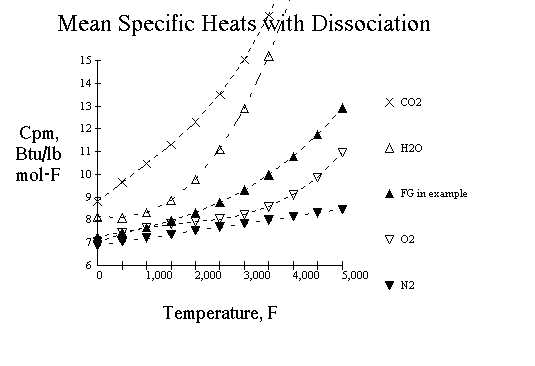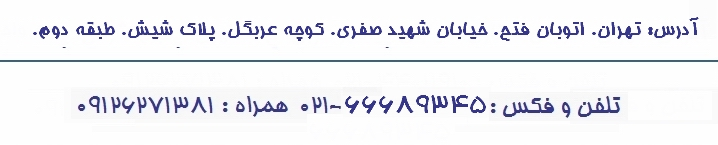اولين سايت تخصصي آموزش و نگهداري و تعميرات ديگ بخار، ديگ آبگرم، ديگ روغن داغ و آموزش نگهداري و طراحي تاسيسات در ايران به صورت کاملا فارسي، و با استناد به آخرين دستاوردهي تکنولوژي ديگ هاي بخار و تاسيسات مربوطه، در جهان.:::| درج آگهي | فروشگاه | | درباره ما | تماس با ما |:::

Flame Temperature Calculator:

Based on article by Carl A. Vancini, in Chemical Engineering, March 22, 1982 Here a simple heat balance serves as the basis for calculating the flame temperature. The increase in enthalpy between the unburned and burned mixtures is set equal to the heat produced by combustion.
Up to a flame temperature of about 2,500 °F, the burned mixture generally includes such ordinary gases as CO2, N2, SO2, H2O, and residual O2 (from excess air). At higher temperatures, CO2 appreciably dissociates to CO and O2; H2O to O2 and OH-; O2 to O-2; H2 to H+ ; N2 to N-3 and NO (produced by N2 and O2) to N-3 and O-2. These dissociation reactions absorb an enormous amount of energy (heat), substantially lowering the flame temperature being calculated.
Heat Balance
The heat balance is calculated as follows: At constant pressure, the heat, Q, required to bring the temperature of one pound of gas from temperature 0 to temperature t is:

 Q = f0t cp dt (1)

The variation of cp with temperature can be approximated by a polynomial, having the obvious advantage of being integrated easily. Using a third-degree polynomial, we can write cp as:

 cp = a + bt + ct2 + dt3 (2)

where, a, b, c, and d are constants that depend on the nature of the gas. Equation (1) thus becomes:

 Q = f0t (a + bt + ct2 + dt3 )dt (3)

Integrating:

 Q = (a + bt/2 + ct2/3 + dt3/4)t (4)

It is customary to call the parenthetic term in Equation (4) the mean specific heat:

 Cmp = a + bt/2 + ct2/3 + dt3 /4 (5)

So Equation 4 can be written as:

 Cmpt = at + bt2/2 + ct2/3 + dt4 /4 (6)

By taking mean specific heats instead of the true ones, we can dispense with integration of Equation (1). Tabulations are available of cp and cpm for many gases (not considering dissociation).
Dissociation
High-temperature dissociation of gases is a reaction that reaches equilibrium. Equilibrium depends on composition, total pressure and temperature. The degree of approach to equilibrium is also time-dependent. Tabulations of equilibrium constants for common dissociation reactions are available.
Hand calculation of the effect of dissociation on the flame temperature is extremely tedious, owing to the large number of reactions involved. For example, in the combustion of acetylene with air, one must solve a set of ten equations for ten unknown partial pressures. Here, polynomial expressions for the equilibrium constants can be used , although with much difficulty.
Justi  gives values of the ratio nU/t (see nomenclature) for several dissociation reactions at various temperatures at 1 atm. Assuming a linear variation of the dissociation effects on temperature with the partial pressure of each dissociating gas, the ratio nU/t can be used to modify the mean specific heat of gases.
The figure illustrates the variation of cpm with temperature, for several gases. This variation takes into account the above approach, which includes the effect of dissociation. The profiles of cpm in this figure are somewhat different from those calculated by applying the modifiers of Justi. Those in the figure use more-recent thermal data and better values of calculated flame temperatures.Limiting the maximum temperature to 5,000°F, a third-degree polynomial can be fitted to the curves of cpm in the figure for CO2, H2O, and O2. For N2, which dissociates appreciably only above 5,000 °F, a second-degree polynomial is satisfactory. The coefficients are shown in Table II.
For transferring heat at a constant pressure:

 Q = [a(t2-t1) + b/2(t2-t1)2 + c/3(t2-t1)3 + d/4(t2-t1) 4]M (6)

where Q is the heat produced by the fuel, M the amount of flue gas produced per pound of fuel, and t1 and t2 the initial and final temperatures, respectively.
M is also equal to the weight of fuel plus comburent (the substance that supports combustion-e.g., air or oxygen). The initial temperature, t1, is that of the fuel and comburent mixture.
The use of Equation (7) allows calculation of the flame temperature, t2, by iteration via a programmable calculator. The result is not, however, to be taken at face value, but rather for comparison. This is because the actual flame temperature is always lower than the calculated one (by any method), for several reasons:
1. There is radiation and conduction to the walls of the chamber in which combustion takes place. Highly turbulent flames usually suffer an appreciable heat loss.
2. Not all of the fuel is burned. Some part may remain with the ash(as the case for solid fuels) or escape combustion in other ways.
3. The combustion space may be of such volume or geometry that there is not enough time for all reactions of combustion and dissociation to reach equilibrium.
In practice, the foregoing factors are usually compensted for by using an empirical coefficient. it is multiplied by the heat of combustion that is determined in the laboratory. Values of this coefficient are only estimates; this is why the flame temperature calculated with any method can only approximate actual values.

 Nomenclature cp True specific heat, Btu/lb-°F cpm Mean specific heat, Btu/lb-°F n Fraction of gas dissociated M Flue gas produced by one pound of fuel, lb/lb Q Heat evolved by fuel during combustion, Btu/lb t Temperature, °F t1 Initial temperature of mixture fuel plus comburent, °F t2 Temperature (average)of flame, °F U Heat absorbed by dissociation reaction, Btu/lb-mol
 Empirical coefficients used in Equation (5). Values account for dissociation Table II Gas a b/2 c/3 d/4 Carbon dioxide 8.83 1.79 X 10-3 -2.63 X 10-7 1.19 X 10-10 Oxygen 7.04 1.0 X 10-3 -4.33 X 10-7 7.8 X 10-11 Water vapor 8.14 -3.1 X 10-4 4.3 X 10-7 8.7 X 10-11 Nitrogen 6.89 3.3 X 10-4 -3.0 X 10-9 Range of validity: 0 to 5,000°F
 N2 + Ar, % vol: CO2 + SO2, % vol: O2, % vol: H2O, % vol: Pounds of Fluegas(wet)/1 lb Fuel, lb/lb: Heat Losses, %: Higher Heating Value of Fuel, Btu/lb: Temperature of Comburent + Fuel, °F: Flame Temperature, °F: a coefficient: b/2 coefficient: c/3 coefficient: d/4 coefficient: Molecular Weight of Fluegas: Useful HHV of Fuel, Btu/lb:

References:
1. Justi, E., and Luder, H., Furshung auf dem Gebide des Ingeniceruesiens, Sept/Oct 1935, pp 210-211
2. Justi, E., Spezifische Warme, Enthalpie, Entropic and Dissoziation technischer Gase, J. Springer Pub., Berlin, 1938, pp 143-151.
3. JANAF Thermochemical Tables, US Dept. of Commerce, Nat. Bur. of Standards, NSRDS-NBS 27. 2nd ed., 1971.
4. Justi, E., op. cit. , pp 130-134.
5. Gaydon, A. G., and Wolfhard, H. G., Flames, Chapman and hall Pub., London, 1979, p. 334.
6. Protero, A., Combustion and Flame, Vol. 13, 1967, p. 399.
7. Justi, E., op. cit. , p. 215.
8. Gaydon, and Wolfhard, op. cit. , pp. 228 and 339.
9. Gas Engineers Handbook, McGraw-Hill Book Co., New York, 1934.
10. Johnson, A. J., and Auth, G. H., (eds), Fuels and Combustion Handbook, McGraw-Hill Book Co., New York, 1950, p. 280.
11. Schnidman, I., (ed), Gaseous Fuel, American Gas Assn., New York, 1948.

»» جهت دریافت اطلاعات کامل در مورد این مقاله و پکیج محاسبات حرارتی با شماره تلفن 09126271381 تماس حاصل فرمایید.

 :::| صفحه اصلي | درج آگهي | فروشگاه | | درباره ما | تماس با ما |:::©کليه حقوق مادي و معنوي اين سايت متعلق به این شرکت است. هر گونه کپي برداري از اطلاعات سايت بدون اطلاع مدير سايت ممنوع و ذکر تمامي مطالب با درج منبع بلامانع است.

منوی اصلی

لیست مقاله ها

لینک های ثابت

 واحد طراحی گروه مشاوره محصولات

اطلاع از به روز رسانی

 عضويت لغو عضويت

جستجو

 وب سایت